# Pressure

The normal force exerted by liquid at rest on a given surface in contact with it is called thrust of liquid on that surface.

The normal force (or thrust) exerted by liquid at rest per unit area of the surface in contact with it is called pressure of liquid or hydrostatic pressure.

If F be the normal force acting on a surface of area A in contact with liquid, then pressure exerted by liquid on this surface is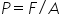(1) Units :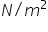Pascal (S.I.) and Dyne/cm2 (C.G.S.)

(2) Dimension :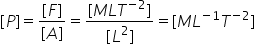(3) At a point pressure acts in all directions and a definite direction is not associated with it. So pressure is a tensor quantity.

(4) Atmospheric pressure : The gaseous envelope surrounding the earth is called the earth's atmosphere and the pressure exerted by the atmosphere is called atmospheric pressure. Its value on the surface of the earth at sea level is nearly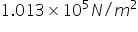Pascal in S.I. other practical units of pressure are atmosphere, bar and torr (mm of Hg)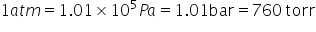The atmospheric pressure is maximum at the surface of earth and goes on decreasing as we move up into the earth's atmosphere.

(5) If Po is the atmospheric pressure then for a point at depth h below the surface of a liquid of density P, hydrostatic

pressure P is given by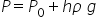(6) Hydrostatic pressure depends on the depth of the point below the surface (h), nature of liquid (P) and acceleration due to gravity (g) while it is independent of the amount of liquid, shape of the container or cross-sectional area considered. So if a given liquid is filled in vessels of different shapes to same height, the pressure at the base in each vessel's will be the same, though the volume or weight of the liquid in different vessels will be different.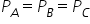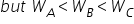(7) In a liquid at same level, the pressure will be same at all points, if not, due to pressure difference the liquid cannot be at rest. This is why the height of liquid is the same in vessels of different shapes containing different amounts of the same liquid at rest when they are in communication with each other.(8) Gauge pressure : The pressure difference between hydrostatic pressure P and atmospheric pressure P0 is called gauge pressure.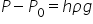Related Keywords